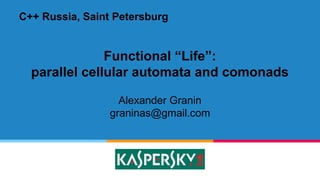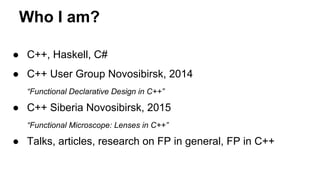Successfully reported this slideshow.

# Functional "Life": parallel cellular automata and comonads

1

ShareYouTube videos are no longer supported on SlideShare×
1 of 35
1 of 35

# Functional "Life": parallel cellular automata and comonads

1

Share

Game of Life built in C++ using functional design (comonads, functional programming, monads). Parallel computations featured by monad-like futures.

Game of Life built in C++ using functional design (comonads, functional programming, monads). Parallel computations featured by monad-like futures.

## More Related Content

### Related Books

Free with a 14 day trial from Scribd

See all

### Related Audiobooks

Free with a 14 day trial from Scribd

See all

### Functional "Life": parallel cellular automata and comonads

1. 1. Functional “Life”: parallel cellular automata and comonads Alexander Granin graninas@gmail.com C++ Russia, Saint Petersburg
2. 2. Who I am? ● C++, Haskell, C# ● C++ User Group Novosibirsk, 2014 “Functional Declarative Design in C++” ● C++ Siberia Novosibirsk, 2015 “Functional Microscope: Lenses in C++” ● Talks, articles, research on FP in general, FP in C++
3. 3. struct Presentation { Functional programming in С++ Functionally designed cellular automation Parallel computation of cellular automation };
4. 4. 4 Functional programming in C++
5. 5. C++ FP Enthusiasts ● Range v3 by Eric Niebler - proposal for C++ Standard Lib ● FTL (Functional Template Library) by Bjorn Aili ● Cat by Nicola Bonelli - Category Theory elements ● Bartosz Milewski ● John Carmack ● … ● <Place your name here>
6. 6. С++ User Group Novosibirsk, 2014 “Functional Declarative Design in C++”
7. 7. С++ Siberia Novosibirsk, 2015 “Functional Microscope: Lenses in C++” auto lens = personL() to addressL() to houseL(); Account account1 = {...}; Account account2 = set(lens, account1, 20); // account2.person.address.house == 20 std::function<int(int)> modifier = [](int old) { return old + 6; }; Account account3 = over(lens, account2, modifier); // account3.person.address.house == 26 Lens 2 Lens 3Lens 1
8. 8. FP elements in C++ ● Lambdas, closures, functions (almost pure) ● std::function<> ● Immutability, POD-types ● Templates - pure functional language ● for_each(), recursion ● C++ Concepts: coming soon...
9. 9. 9 Simple 1-dimensional 3-state CA ● 1 dimension ● 3 states: A (“Alive”), P (“Pregnant”), D/space (“Dead”), A A A A A A P P A A A 1 gen P 2 gen A A A 3 gen A A A A 4 gen A P A 5 gen A A A 6 gen A A A A 7 gen A P A
10. 10. template <typename T> struct Universe { std::vector<T> field; int position; }; typedef char Cell; const Cell Pregnant = 2; const Cell Alive = 1; const Cell Dead = 0; Universe<Cell> A A A A Universe<T>: Pointed array Universe<Cell> u; u.field = {D, A, A, D, A, A, D}; u.position = 3;
11. 11. Immutable shift A A A A Universe<Cell> left (const Universe<Cell>& u) { Universe<Cell> newU { u.field, u.position - 1 }; if (u.position == 0) newU.position = u.size() - 1; return newU; } Universe<Cell> right (const Universe<Cell>& u); A A A A A A A A shift to right shift to left
12. 12. Observing: shift and extract A A A A Cell extract(const Universe<Cell>& u) { return u.field[u.position]; } Universe<Cell> u = {...}; Cell cur = extract (u); Cell r = extract (right (u)); Cell rr = extract (right (right (u))); Cell l = extract (left (u)); Cell ll = extract (left (left (u))); D A A A A shift to left shift to left extract
13. 13. Rule: observe and reduce A A A A P Cell rule(const Universe<Cell>& row) { // extract l, ll, cur, r, rr here if (isA(l) && isA(r) && !isAorP(cur)) return Pregnant; // ... more rules here return Dead; }
14. 14. Applying rule: extend, extract Cell rule (Universe<Cell> row); Universe<Cell> extend ( std::function<Cell(Universe<Cell>)> f, const Universe<Cell>& u); Cell extract(const Universe<Cell>& u); A A A A P P
15. 15. Step: duplicate, (for_each: extend, extract) A A A A A A A A A A A A A A A A A A A A A A A A A A A A A P A A P A Cell rule (Universe<Cell> row); Universe<Cell> extend ( std::function<Cell(Universe<Cell>)> f, const Universe<Cell>& u); Universe<Universe<Cell>> duplicate (const Universe<Cell>& u); Universe<Cell> left (const Universe<Cell>& u); Universe<Cell> right (const Universe<Cell>& u);
16. 16. Universe<Cell> r1; r1.position = 0; r1.field = {D, D, D, P, D, D, D}; Universe<Cell> r2 = stepWith(rule(), r1); Universe<Cell> r3 = stepWith(rule(), r2); Universe<Cell> ( std::function<Cell(Universe<Cell>)> f, const Universe<Cell>& u) { return extend(f, ut); } Step
17. 17. 17 Generic functional approach #define UT Universe<T> #define UUT Universe<Universe<T>> template <typename T> T rule (const UT& u) template <typename T> UT left (const UT& u) template <typename T> UT right (const UT& u)
18. 18. Generic extract template <typename T> T extract(const UT& u) { return u.field[u.position]; }
19. 19. Generic extend template <typename T> UT extend ( const func<T(UT)>& f, const UT& u) { UUT duplicated = duplicate (u); return { map(f, duplicated.field), u.position }; }
20. 20. Generic map template<typename A, typename B, template <class ...> class Container> Container<B> map ( const std::function<B(A)>& f, const Container<A>& va) { Container<B> vb; std::transform(va.begin(), va.end(), std::back_inserter(vb), f); return vb; }
21. 21. Generic duplicate const std::function<UT(UT)> leftCreator = [](const UT& u) {return left(u); }; const std::function<UT(UT)> rightCreator = [](const UT& u) {return right(u); }; template <typename T> UUT duplicate (const UT& u) { return makeUniverse (leftCreator, rightCreator, u); }
22. 22. Generic makeUniverse template <typename T> UUT makeUniverse ( const std::function<UT(UT)>& leftCreator, const std::function<UT(UT)>& rightCreator, const UT& u) { std::vector<UT> lefts = tailOfGen(u.position, leftCreator, u); std::vector<UT> rights = tailOfGen(u.size() - u.position - 1, rightCreator, u); std::vector<UT> all; all.swap(lefts); all.push_back(u); all.insert(all.end(), rights.begin(), rights.end()); return { std::move(all), u.position }; }
23. 23. extract + duplicate + extend = comonad template <typename T> T extract (const UT& u) template <typename T> UT extend ( const func<T(UT)>& f, const UT& u) template <typename T> UUT duplicate (const UT& u)
24. 24. 24 Parallel computations in FP Container<B> map ( const std::function<B(A)>& f, const Container<A>& va); Container<B> mapPar ( const std::function<B(A)>& f, const Container<A>& va);
25. 25. mapPar template <typename A, typename B, template <class ...> class Container> Container<B> mapPar ( const std::function<B(A)>& f, const Container<A>& va) { Container<std::future<B>> pars = map(par(f), va); std::future<Container<B>> pRes = joinPars(pars); return pRes.get(); }
26. 26. template <typename A, typename B> std::function<std::future<B>(A)> par( const std::function<B(A)>& f) { return [=](const A& a) { return std::async(std::launch::async, [=]() { return f(a); } ); }; } par
27. 27. template <typename B> std::future<std::vector<B>> joinPars( std::vector<std::future<B>>& pars) { return std::async(std::launch::async, [&]() { std::vector<B> bs; bs.reserve(pars.size()); for (auto& it : pars) bs.push_back(it.get()); return bs; }); } joinPars
28. 28. 28 Parallel Game of Life benchmark ● 2 dimensions ● 2 states: A (“Alive”), D/space (“Dead”), // Pointed array of pointed arrays typedef Universe<Cell> LifeRow; typedef Universe<LifeRow> LifeField;
29. 29. A little bit harder... #define UT Universe<T> #define UUT Universe<Universe<T>> #define UUUT Universe<Universe<Universe<T>>> #define UUUUT Universe<Universe<Universe<Universe<T>>>> template <typename T> UUUUT duplicate2 (const UUT& u) template <typename T> UUT extend2 ( const func<T(UUT)>& f, const UUT& u) template <typename T> T extract2 (const UUT& u)
30. 30. extend vs extend2 template <typename T> UT extend ( const func<T(UT)>& f, const UT& u) { UUT duplicated = duplicate (u); return { map (f, duplicated.field), u.position }; // == fmap (f, duplicated.field) } template <typename T> UUT extend2 ( const func<T(UUT)>& f, const UUT& uut) { UUUUT duplicated = duplicate2 (uut); return fmap2 (f, duplicated); }
31. 31. fmap2 template <typename T> UUT fmap2 ( const func<T(UUT)>& f, const UUUUT& uuut) { const func<UT(UUUT)> f2 = [=](const UUUT& uuut2) { UT newUt; newUt.position = uuut2.position; newUt.field = map (f, uuut2.field); return newUt; }; return { map (f2, uuut.field), uuut.position }; // parallelization: map -> mapPar }
32. 32. Game of Life benchmark Field side Sequential Parallel (milliseconds) 50 484 283 100 3900 2291 150 12669 8005 200 30278 19415 auto l1 = gliderLifeHuge(); QBENCHMARK { auto l2 = stepWith(rule, l1); QVERIFY(l2.size() == HugeSize); }
33. 33. Game of Life on comonads, C++ ● Highly experimental ● Sequential, async and parallel GoL ● Simple 1D 3-state CA ● Functional design ● https://github.com/graninas/CMLife ● Клеточные автоматы и комонады, by Hithroc Mehatoko
34. 34. Thank you! Alexander Granin graninas@gmail.com Questions? C++ Russia, Saint Petersburg